## research papersJOURNAL OFSYNCHROTRONRADIATION
ISSN: 1600-5775
Volume 26| Part 3| May 2019| Pages 819-824

## Time-resolved X-ray reflection phases of the nearly forbidden Si(222) reflection under laser excitation

aNational Synchrotron Radiation Research Center, Hsinchu 300, Taiwan, bDepartment of Physics, National Tsing Hua University, Hsinchu 300, Taiwan, and cCenter for Measurement Standards, Industrial Technology Research Institute, Hsinchu 300, Taiwan
*Correspondence e-mail: slchang@phys.nthu.edu.tw

(Received 7 August 2018; accepted 12 March 2019; online 12 April 2019)

The covalent electron density, which makes Si(222) measurable, is subject to laser excitation. The three-wave Si(222)/(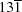) diffraction at 7.82 keV is used for phase measurements. It is found that laser excitation causes a relative phase change of around 4° in Si(222) in the first 100 ps of excitation and this is gradually recovered over several nanoseconds. This phase change is due to laser excitation of covalent electrons around the silicon atoms in the unit cell and makes the electron density deviate further from the centrosymmetric distribution.

### 1. Introduction

X-ray diffraction or scattering by solids under external excitations, such as ultrafast laser excitation and high-field pumping, are currently important trends in understanding the time-dependent physical properties and dynamic structures of solids on different time scales (Lindenberg et al., 2005; Fritz et al., 2007; Hillyard et al., 2007; Johnson et al., 2009; Papalazarou et al., 2012). Based on this, time-resolved experiments, pump–probe techniques and free-electron laser related experiments have recently received much attention (Johnson et al., 2008; Clark et al., 2013; Hayashi et al., 2006). Some important problems and phenomena have been studied covering different time domains. Here, we focus on the longstanding phase problem for condensed matter and crystalline materials and try to link the relative phase change of a crystal under laser-beam excitation.

Phase is a relative physical quantity, like potential, which plays a very important role in many physical systems. In the case of X-ray diffraction and scattering from condensed matter, measuring the phase may provide information on the static and dynamic structure of a material system. Strictly speaking, the phase' is meant to be the phase of the usual structure factor' of a given reflection or scattering. It is known that the scattered or diffracted intensity of a single reflection provides no phase information on the structure factor, because the intensity is only proportional to the product of the structure factor and its complex conjugate. The phase information is therefore lost in the intensity measurement. This fact constitutes the so-called X-ray phase problem in diffraction and scattering experiments (Lipscomb, 1949; Hauptman & Karle, 1953; Giacovazzo, 2002; Giacovazzo et al., 1992). Recent development of multiple diffraction techniques has shown that the phase of a structure-factor triplet can be determined quantitatively from the intensity of three-wave diffraction in a crystalline material system under resonance or non-resonance conditions (Miyake & Kambe, 1954; Ewald & Héno, 1968; Colella, 1974; Post, 1977; Chapman et al., 1981; Chang, 1982; Juretschke, 1982; Hümmer & Weckert, 1990; Chang et al., 1991; Weckert et al., 1993; Weckert & Hümmer, 1997; Chang et al., 1998; Shen, 1998, 1999; Stetsko et al., 2001; Morelhão & Kycia, 2002). As an initial experiment, we have selected the well known silicon crystal and investigated the phase change due to laser excitation in a few hundred picoseconds in relation to the redistribution of the averaged covalent electron density under quasi-thermodynamic equilibrium.

Silicon is the most commonly used semiconductor. It crystallizes in the diamond structure in space group Fd3m. It is known that silicon, a centrosymmetric crystal, has a face-centred cubic (diamond cubic) structure. The structure factor of a reflection (hkl) with Miller indices h, k, l even–odd mixed or h, k, l an odd multiple of 2, is zero, which is called the forbidden reflection because no diffracted intensity is expected. However, in the literature the space-group forbidden (222) reflection of the diamond structure does have measurable diffraction intensity (Bragg, 1921; Renninger, 1960; Roberto & Batterman, 1970; Fujimoto, 1974) because of X-ray scattering from covalent electrons donated by two adjacent diamond atoms along the  direction. For silicon there are eight Si atoms in the unit cell. These form tetrahedral bonds, and each atom sees a bond (and nearest neighbour) in one direction and a hole' in the opposite direction along any (111) axis (Roberto & Batterman, 1970).

If an ultrafast laser beam excites silicon along the  direction, it is suspected that the Si atoms would spend more of their time displaced towards the holes than towards the bonds. The relative positions of the average covalent electron densities would then be changed. In other words, the symmetry of the electron density of unperturbed silicon would be destroyed by laser excitation. Specifically, the phase of the structure factor F(222) in the normal situation would vary (Roberto et al., 1974). The question then arises: what are the amplitude and phase of F(222) in terms of time?

To answer this question, we designed a time-resolved experiment, measuring the phase change of Si with and without laser excitation. The Si(000, 222,) [abbreviated as Si(222)/()] three-wave diffraction technique was adopted to measure first the phase of the structure-factor triplet F()F(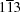)/F(222) involved in the three-wave diffraction, and then the phase of the (222) reflection, assuming that the phase changes of relatively strong reflections, like () and (), due to covalent electrons are negligibly small. The Si(111) is pumped by a Ti:sapphire laser and probed by synchrotron X-rays. The variations in the Si(222) phase and intensity with respect to unperturbed normal silicon are measured by X-rays.

### 2. Experimental

The experiments were carried out on beamline TPS 09A of the newly established Taiwan Photon Source (TPS) at the National Synchrotron Radiation Research Center (NSRRC). Fig. 1shows the layout of the experimental setup. The storage ring is operated in single-bunch mode with a beam current of 2 mA. The frequency of the X-rays is 578 kHz and the effective time resolution of the whole system is around 100 ps as measured using a standard sample of Si(004). The incident X-rays generated by a 3 m in-vacuum undulator are directed by a double-crystal Si(111) monochromator (DCM) and a horizontal focusing mirror (HFM), and collimated onto an Si single crystal mounted at the centre of a nine-circle diffractometer. A Ti:sapphire laser beam (laser wavelength 800 nm, frequency 1 kHz, pulse width 35 fs), synchronized with the X-rays, impinges on the crystal sample at 30° relative to the incident X-ray beam direction. During single-bunch operation, the time interval of the X-ray pulses is around 1.73 µs. The effective photon flux of the X-rays used is 1.1 × 105 and the energy power density of the laser on the sample surface is 1.45 mJ cm−2 pulse−1. An avalanche photodiode (APD0008; FMB Oxford) and a Pilatus 200 K area detector (Dectris) were used as X-ray detectors. The response times of the APD0008 and Pilatus 200 K are around 4 ns and 115 ns (Ejdrup et al., 2009), respectively. An Si(113) beam extender (BE) in an asymmetric diffraction geometry was used in front of the detector to increase the X-ray beam size about twelve­fold to avoid saturation on the area detector.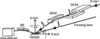Figure 1 A schematic diagram of the experimental setup

A  oriented silicon crystal, without the laser beam on, was aligned for the (222) reflection by adjusting the theta angle, θ222, for the photon energy of 7.82 keV. The three-wave (000)(222)() diffraction is conducted by rotating the crystal around the reciprocal-lattice vector  (the azimuth ϕ scan), without disturbing the (222) reflection, to bring the additional reflection, (), also satisfying the Bragg condition simultaneously. The coherent interaction among the incident (000), the primary (222) and the secondary () gives rise to the intensity variation, monitored by the X-ray detector at 2θ222, on the intensity background of the (222) reflection as a function of ϕ. Fig. 2(a) shows the measured multiple diffraction pattern, the so-called Renninger scan (ϕ scan; Renninger, 1937) of Si(222) on a semi-logarithmic scale to show the weak asymmetry near the (222) intensity background. The mirror symmetry of the pattern occurs at ϕ = 60°, at which the [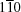] is aligned with the incident X-ray beam. The three-wave (000)(222)() diffraction, denoted (222)/(), is located at ϕ = 47.41° and its diffraction profile on a linear scale is shown in Fig. 2(b). It should be noted that at 7.82 keV there are no other multiple diffractions appearing near ϕ = 47.41°. As is well known, the peak profile shows an intensity asymmetry with respect to ϕ = 47.41°, i.e. the intensity first decreases and then increases, indicating the phase sum (Chang, 1982) at the IN position (when the reciprocal-lattice point ofis moving towards the surface of the Ewald sphere). The triplet phase δ3 =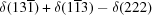= 0°, where () is the coupling between (222) and (), in agreement with the centrosymmetric nature of Si. Fig. 2(c) shows the rocking curve for the Si(222) two-wave case.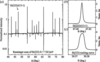Figure 2 (a) A Renninger scan of Si(222) at E = 7.82 keV near ϕ = 60°. (b) The three-wave Si(222)/() diffraction profile. (c) An Si(222) rocking curve.

### 3. Three-wave diffraction with laser-beam excitation

The Si crystal is then excited by the laser beam with an energy density of 1.45 mJ cm−2 pulse−1. After reaching thermodynamic equilibrium (as a result of heating by the laser illumination) and re-alignment of the crystal diffraction conditions to compensate for the heating effect, the pump–probe experiment starts. The time when the laser beam hits the Si crystal is marked as t0 = 0, and the probing X-ray beam is used at t0 + Δt to measure the phase change via three-wave diffraction. Because the time scales of synchrotron sources are in tens to hundreds of picoseconds, thermal and structural effects dominate (Sundaram & Mazur, 2002). Fig. 3(a) shows the measured intensity profiles at Δt equal to −200 and 100 ps for three-wave (222)/() diffraction. From the overall intensities shown in the insets of Figs. 3(a) and 3(b), nearly identical profiles are obtained. However, if we show the intensity asymmetry (the phase profiles) relative to the Si(222) baseline intensity (the dashed black lines), the two measured curves show clear differences. To estimate the phase difference, we employ the dynamical theory of X-ray diffraction (Authier, 2001) for multiple diffraction (Chang, 2004) to calculate the intensity profiles for comparison with measured phases at different Δt.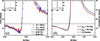Figure 3 (a) Measured Si(222) intensities of Si(222)/) for 200 ps before (blue curve) and 100 ps after (red curve) the laser beam was turned on. (b) Calculated intensity profiles for panel (a).

The simulated multiple-wave diffraction profiles shown in Fig. 3(b) are based on the algorithm for solving multiple-wave dynamical X-ray diffraction equations in the Cartesian coordinates representation (Stetsko & Chang, 1997), where the fundamental equations of wavefields are expressed in Cartesian coordinates. The corresponding wavefield solutions can easily be found from the eigenvalue eigenvectors, with minimal effects from the variation of polarization factors due to the change in incidence conditions. In the theoretical simulations, the diffraction geometry of the multi-wave diffraction (000)(222)(), the boundary conditions and the structure factors of the involved reflections are considered as the input data for the dynamical calculations. Since the electron density of Si is distorted by covalent effects, the conventional structure factor calculated for centrosymmetric Si is not suited to the current situation. Instead, multipole refinement methods are adopted to obtain the electron-density distribution in the unit cell of silicon including covalent effects (Hansen & Coppens, 1978; Pietsch et al., 1986; Roberto & Batterman, 1970), using the software package XD2016 (Volkov et al., 2016).

Fig. 4(a) is the calculated electron-density map of Si using multipole refinement methods. The contours in the different shades from light red to crimson correspond to electron densities of 0.23, 0.55 and 3 e Å−3. A cross section of Fig. 4(a) along the a = b direction is shown in Fig. 4(b). The electron density close to the core of the silicon atoms is around 5000 e Å−3. The cut-off electron density is 1.2 e Å−3, marked as yellow images in Fig. 4(b), to emphasize the electron-density distribution between Si atoms. It is obvious that the shape of the electron density of each silicon atom deviates from spherical symmetry, and this significant deviation of electron density is shown by holes' in a darker colour and the `bond' in a lighter colour observed along the  direction.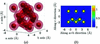Figure 4 (a) A calculated electron-density map of Si using multipole refinement methods. (b) A cross section of panel (a) along the a = b direction.

The retrieved electron density is then used to calculate the structure factors in the simulations. When the effect of the covalent electron is considered, the diffraction behaviour of a silicon crystal is quite different from the conventional treatment of silicon (Pietsch et al., 1986), especially for weak and/or forbidden reflections. For instance, using the calculated results with a spatial resolution of 0.0125 Å from the multipole refinement, the amplitude and phase of the structure factor of Si(222), F222 =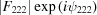, are |F222| = 3.252 electrons and ψ222 = 57.6° in simulations when considering covalent electron effects. In contrast, the amplitude and phase are both zero in the conventional treatment because of the centrosymmetric structure. Table 1shows a comparison of the structure factor amplitudes and phases of reflections from silicon with and without covalent electron effects. Hence, in simulations for multi-wave diffraction profiles using the dynamical theorem, we assume that the amplitude of the structure factor remains constant, |F222| = 3.252 electrons, but with a varying phase ψ222, resulting from laser excitation time Δt. When the simulated three-wave profile is in agreement with the measured profile, the phase is then correctly determined. In practice, the effects of the divergence of the incident beam need to be considered in matching the simulation and the measured profiles. That is, the simulated profile is convoluted with θ and ϕ, the divergences of the incident beam in the vertical direction θ and the horizontal direction ϕ, respectively. The convolution with θ is taken over the Darwin width of Si(222). A Gaussian function, y = exp[−(Δϕ)2/2w2], is used for the convolution over ϕ, where w is the horizontal divergence of the incident X-ray beam.

 Table 1Calculated structure-factor amplitudes (electrons) and phases (°) with and without covalent electron effects for silicon (E = 7.82 keV), ignoring the absorption effect
Reflection |F|covalent |F|conventional ψcovalent ψconventional
000 113.2 114.2 0.0 0
111 62.4 60.4 −177.6 π
220 71.0 69.7 179.9 π
311 46.0 45.8 177.4 π
222 3.2 0.0 57.6 0
400 59.1 58.5 −179.7 π
331 40.6 39.2 3.5 0
422 52.0 50.9 0.6 0
333 34.6 34.2 −2.3 0
511 35.0 34.2 3.8 0
440 45.5 44.6 0.0 0
442 2.5 0.0 −96.2 0
622 2.6 0.0 −92.2 π

Fig. 3(b) shows the simulated diffraction profiles. The phases, ψ222, are 56.6° and 60.6°, which correspond to the measurements of Δt at −200 ps and 100 ps. The measured phase before the laser excitation, ψ222, equals 56.9°. The relative phase changes, Δψ222 = ψ222 − 56.9°, are −0.3° and 3.7°, approximately equal to 0° and 4° within experimental error. These results are in fair agreement with the measured profiles shown in Fig. 3(a).

Lattice distortions of crystals usually occur due to laser excitations with a high laser fluence. For example, an observation of an angular shift of more than 0.1 arcsec for (111) with a laser fluence of 382 mJ cm−2 pulse−1 has been reported (Hayashi et al., 2006). In order to check the diffraction conditions with and without laser excitation, the diffraction intensities of (111) and (222) were monitored as a function of Δt. The rocking curves of (111) and (222) without laser excitation were measured first, and then the incident conditions were fixed at the angles with half of the maximum intensities on the rocking curves of (111) and (222) to monitor the intensity perturbations due to the laser excitation. If the rocking curves are distorted by the laser excitation, such as peak shifting or diffraction power dropping, the monitored intensities are changed. In the (111) case, we observed that the peak position of the rocking curve remained unchanged and the diffraction power dropped after laser excitation. The intensity monitoring of (111) as a function of Δt is shown as a red line in Fig. 5(a). The edge of the intensity drop also indicates the zero time (Δt = 0) when the laser pulse and the X-ray pulse illuminate the sample simultaneously. In the case of (222), the deviation of the rocking curve after laser excitation is unobservable and the intensity monitoring is shown as a blue line in Fig. 5(a). These results for (111) and (222) not only determine the zero time but also indicate that the lattice distortion during the experiments is negligibly small because the applied laser fluence of 1.45 mJ cm−2 pulse−1 in our experiments is low.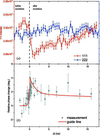Figure 5 (a) Si(111) and Si(222) intensities versus X-ray probed time Δt. (b) The measured relative phase change (in °).

In Fig. 5(b), as is indicated, the relative phase-changing tendency rises sharply from approximately Δψ222 = 0° to nearly Δψ222 = 4° within 100 ps after laser excitation. Then in 15 ns, the relative phase change is gradually recovered.

The fitting results also show that the beam divergence is equal to 134 µrad in the horizontal direction. The vertical beam divergence is considered around 25 µrad from the measurement of the undulator. Significantly, the relative phase change in these experiments, Δψ222, increases after laser excitation. This tendency indicates that the electron-density distribution in the unit cell tends to deviate from centrosymmetry, because the phase in a centrosymmetric cubic structure is nearly 0°, with acute profile asymmetry. When the noncentrosymmetry (covalent electrons) comes into play, the phase increases and the profile asymmetry decreases (without laser beam excitation). When the laser is used, the phase is further increased and the profile asymmetry decreased again.

### 4. Conclusions

In conclusion, we have reported a time-resolved X-ray reflection phase measurement of the nearly forbidden Si(222) reflection under laser excitation, which shows that the relative phase change is around 4° in the first 100 ps after laser excitation and gradually returns to its normal situation in a few nanoseconds. For a much more detailed investigation of the dynamic behaviour of phase changes in the regime of tenths of femtoseconds, a better probing source like a free-electron laser is needed.

### Acknowledgements

The authors are indebted to the NSRRC and the Ministry of Science and Technology (MOST) for financial support. Assistance from the Beamline Group 09A and the Machine Operation Group for single-bunch operation is also gratefully acknowledged. Special thanks go to SPring-8 for the use of BL12XU at the beginning of this study.

### ReferencesJOURNAL OFSYNCHROTRONRADIATION